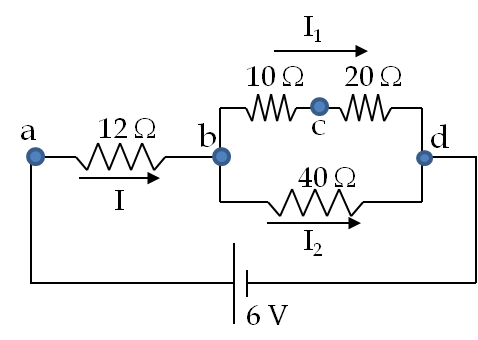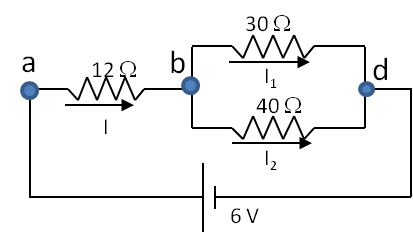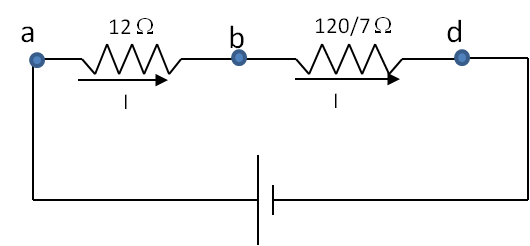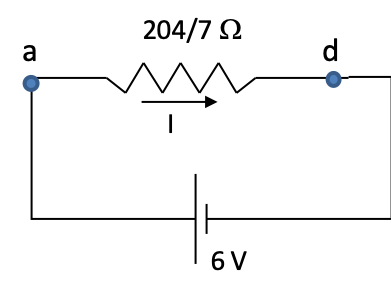## Section36.4Series-Parallel Method

Certain complex circuits can be simplified by a method that uses only the results of resitors in series and in parallel. They provide good examples for deeping your understanding of the series and parallel circuits. We will illustrate this method with an example below.

The basic idea of series-paralllel method is to successively use equivalent resistance rules for series and parallel resistors in a sequence of steps.

1. First we replace all the resistors in series by their equivalent resistances. This step usually leaves some resistors in parallel.
2. Then we replace the resistors that are in parallel by their equivalent resistances. This step generates new resistors in series, which can then be replaced by their equivalent resistances.
3. Continue, if necessary, Item 1, and, if necessary, followed by Item 2, until we simplify the circuit to one net equivalent resistor connected to one source. If at any point, we have more than one resistor remaining and we cannot decide if two or more resistors are in series or in parallel, the process tops with failure of method. You will need more advanced method in those cases.
4. Suppose our process succeeds in simplifying the circuit to one resitor across a source. The one-equivalent-resistor circuit is solved for the ovrall current.
5. The calculated current is used in a backward steps to find potential drops and currents in previously simplifield circuits until all currents and potential drops have been computed.

The series-parallel method does not always work. For instance, if a circuit has a bridge from one branch to another, it becomes impossible to label resistors into series or parallel categories. For these more complicated circuits, you will learn more general method, called Kirchoff's rules in a later section.

Consider four resistors connected to a voltage source as shown in Figure 36.4.2. Find current through each resistor.

Hint

Study the solution.

$\phi_d= 0;$ $\phi_a = 6\ \text{V};$ $\phi_b = \dfrac{60}{17}\ \text{V};$ $\phi_c = \dfrac{40}{17}\ \text{V}.$ $I_1 = \dfrac{2}{7}\ \text{A};$ $I_2 = \dfrac{3}{34}\ \text{A};$ $I = \dfrac{7}{34}\ \text{A}.$

Solution

To find current through the resistors, we need potential drop across each. Therefore, we start with labeling the node potential points on the circuit as shown in the figure.As explained above, the node points represent points of unique electric potential values. There are four nodes in the given circuit, which are labeled $a\text{,}$ $b\text{,}$ $c\text{,}$ and $d\text{.}$ Node $d$ is connected to the negative of the source and is usually set zero volt as reference, $\phi_d = 0\text{.}$ Since voltage of the battery is 6 volts, the potential at $a$ is 6 V, that is, $\phi_a = 0\text{.}$ Now, we follow step-by-step procedure of simplifying the circuit to find the potentials at $b$ and $c\text{,}$ viz., $\phi_b$ and $\phi_c\text{,}$ respectively.

Step 1:

If any resistors in series, replace them by their equivalent resistors. Here only $10\ \Omega$ and $20\ \Omega$ are in series. Therefore, we replace them by a $30\text{-}\Omega$ resistor. It is best to keep the same labels here as in the previous diagram.Step 2:

If any resistors in parallel, replace them by equivalent resistors. Here only 30 $\Omega$ and 40 $\Omega$ are in parallel. Therefore, we replace the combination by their equivalent resistance, which is $\dfrac{120}{7}\ \Omega\text{.}$Step 3:

After replacing the parallel resistors by $\dfrac{120}{7}\ \Omega\text{,}$ we find that it is in series with the $12\text{-}\Omega$ resistor. Therefore current through the $\dfrac{120}{7}\ \Omega$ must be same as that goes through the $12\text{-}\Omega$ resistor. Now the two resistors in series can be replaced by a $\dfrac{204}{7}\text{-}\Omega$ resistor resulting in a really simple circuit. The current through the $\dfrac{204}{7}\text{-}\Omega$ resistor must be same as the $12\text{-}\Omega$ or $\dfrac{120}{7}\text{-} \Omega$ resistor since it is replacing resistors in series.Step 4:

Now, we can use Ohm's law to find the current through $\dfrac{204}{7}\text{-}\Omega$ resistor.

\begin{equation*} I = \dfrac{6V}{(204/7)\Omega} = \dfrac{7}{34}\ \text{A}. \end{equation*}

Step 5:

We then trace our path backwards towards the original circuit. The current through the $12\text{-}\Omega$ resistor is also $7/34\text{ A}\text{.}$ Therefore, potential drop across the $12\text{-}\Omega$ resistor is

\begin{equation*} \phi_a-\phi_b = \dfrac{7}{34}\ \text{A} \times 12\ \Omega = \dfrac{42}{17}\ \text{V}. \end{equation*}

The potential drop across the $\dfrac{120}{7}\text{-} \Omega$ resistor is $(60/17)\text{V}\text{.}$

\begin{equation*} \phi_b-\phi_d = \dfrac{7}{34}\ \text{A} \times \dfrac{120}{7}\ \Omega = \dfrac{60}{17}\ \text{V}\ \ \Longrightarrow\ \phi_b = \dfrac{60}{17}\ \text{V}. \end{equation*}

Going back one more step we find that potential drop across the $30\text{-}\Omega$ resitor is same as that across the $\dfrac{120}{7}\text{-}\Omega$ resistor we worked out. Hence, current through the $30\text{-}\Omega$ resistor is simply

\begin{equation*} I_1 = \dfrac{(60/17)\ \text{V}}{30\ \Omega} = \dfrac{2}{17}\ \text{A} \end{equation*}

Therefore, voltage $\phi_c$ is obtained from the potential drop across the $20\text{-} \Omega$ resistor as given by

\begin{equation*} \phi_c - \phi_d = \left( \dfrac{2}{17}\ \text{A} \right) \times 20\ \Omega = \dfrac{40}{17}\ \text{V}. \end{equation*}

Since $\phi_d = 0\text{,}$ we obtain $\phi_c = \dfrac{40}{17}\ \text{V}\text{.}$ We can use the node voltages to obtain the current through various resistors. Alternately, since the total current $I$ was split into $I_1$ and $I_2\text{,}$ we can find $I_2$ by subtracting $I_1$ from $I\text{.}$

\begin{equation*} I_2 = I - I_1 = \dfrac{7}{34}\ \text{A} - \dfrac{2}{17}\ \text{A} = \dfrac{3}{34}\ \text{A} \end{equation*}